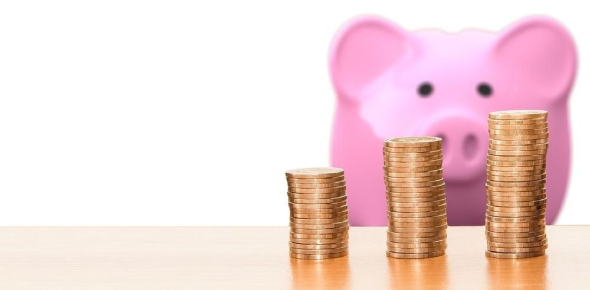# MCQ On Macroeconomics: Trivia Test! Quiz

38 Questions | Attempts: 329
ShareSettings.

• 1.
As the part of a person's wealth that can be readily used for transactions of buying items.
• A.

Currency

• B.

Money supply

• C.

Medium of exchange

• D.

Checking deposits

• E.

Money

• 2.
The level of income or real GDP at which the 45-degree line and the expenditure line cross; also called the equilibrium income.
• A.

Marginal propensity to consume

• B.

Expenditure line

• C.

Spending balance

• 3.
Something of value owned by a person or firm.
• A.

Asset

• B.

Productivity

• C.

Liability

• D.

Unit of account

• E.

Open market operation

• 4.
As labor grows you'll be able to produce more, but at a lower rate.
• A.

• B.

Productivity

• C.

Demand shock

• D.

Velocity

• E.

Diminishing returns

• 5.
The growth rate of productivity = 1/3 (growth rate of capital per hr of work) + (growth rate of technology) OR   Growth rate of technology = growth rate of productivity - 1/3 (growth rate of capital per hour of work)
• A.

Target inflation rate

• B.

Aggregated demand

• C.

Growth accounting formula

• D.

Federal funds rate

• E.

Reserves

• 6.
The discovery of new knowledge or a new principle.
• A.

Innovation

• B.

Invention

• C.

Diffusion

• D.

Disinflation

• E.

Re inflation

• 7.
The central bank's goal for the average rate of inflation over the long run.
• A.

Federal reserves system

• B.

Quantity equation of money

• C.

• D.

Target inflation rate

• E.

Marginal propensity to consume

• 8.
The buying or selling of bonds by the central bank.
• A.

Abc

• B.

Federal open market committee

• C.

Federal reserves system

• D.

Federal funds rate

• E.

Open market operation

• 9.
Something of value that a person/firm owes to someone else.
• A.

Assets

• B.

Liability

• C.

Reserves

• 10.
Something that will allow purchasing power to be carried from one person to the next
• A.

Productivity

• B.

Unit of account

• C.

Bank

• D.

Store of value

• 11.
The equation relating the price level and GDP to the quantity of money and the velocity of money. (Quantity of money) (velocity) = (price level) (real GDP)
• A.

Spending balance

• B.

Quantity equation of money

• C.

Monetary policy rule

• D.

Medium of exchange

• 12.
The fraction of a banks deposits that is required to hold at the federal reserves
• A.

Store of value

• B.

Checking deposits

• C.

Required reserve ratio

• D.

Quantity theory of money

• E.

Inflation

• 13.
The interest rate on overnight loans between banks.
• A.

Federal funds rate

• B.

Required reserve ratio

• C.

Reserves

• D.

Open market operation

• E.

Consumption function

• 14.
Coins & paper money.
• A.

Money

• B.

Store of value

• C.

Assets

• D.

Bank

• E.

Currency

• 15.
Output per unit of labor (Y/L)
• A.

Diminishing returns

• B.

Technological change

• C.

Growth accounting formula

• D.

Productivity

• 16.
Innovation that creates something brand new
• A.

Invention

• B.

Innovation

• C.

Diffusion

• D.

Disinflation

• E.

Demand shock

• 17.
"practice makes perfect"
• A.

Money supply

• B.

Open market operation

• C.

Expenditure line

• D.

Learning by doing

• 18.
New knowledge is brought into application with a new product
• A.

Invention

• B.

Innovation

• C.

Diffusion

• 19.
A line showing a negative relationship between inflation and the aggregated quantity of goods/services
• A.

Expenditure line

• B.

• C.

• D.

Medium of exchange

• 20.
A flat line showing the level of inflation in the economy at a given point in time. It shifts up when real GDP is greater than potential
• A.

• B.

Federal funds rate

• C.

• D.

Expenditure line

• E.

• 21.
A component of the Keynesian Theory, ____ represents the proportion of an aggregate raise in pay that's spent on the consumption of goods/services
• A.

Marginal propensity to consume (MPC)

• B.

• C.

• D.

Quantity equation of money

• 22.
A slowing in the rate of price inflation. ____ is used to describe instances when the inflation rate has reduced marginally over the short term.
• A.

Price shock

• B.

Demand shock

• C.

Disinflation

• D.

Reinflation

• E.

Inflation

• 23.
A condition of slow economic growth and relatively high unemployment. Occurs when the economy isn't growing, but prices are
• A.

Disinflation

• B.

Reinflation

• C.

Inflation

• D.

Invention

• E.

Stagflation

• 24.
A general decline in prices, often caused by a reduction in the supply of money or credit. Deflation in government, personal or investment spending. Can increase unemployment => can then lead to economic depression.
• A.

Opperation

• B.

Disinflation

• C.

Stagflation

• D.

Deflation

• E.

Innovation

• 25.
An item that people are willing to accept as payment for what they are selling because they in turn can use it to pay for something else that they want
• A.

Currency

• B.

Medium of exchange

• C.

Money

• D.

Money supply

## Related TopicsBack to top
×

Wait!
Here's an interesting quiz for you.